A Generalized Linked List L, is defined as a finite sequence of n>=0 elements, l1, l2, l3, l4, …, ln, such that li are either atom or the list of atoms. Thus
L = (l1, l2, l3, l4, …, ln)
where n is total number of nodes in the list.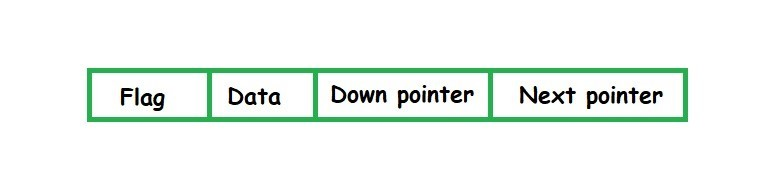To represent a list of items there are certain assumptions about the node structure.

• Flag = 1 implies that down pointer exists
• Flag = 0 implies that next pointer exists
• Data means the atom
• Down pointer is the address of node which is down of the current node
• Next pointer is the address of node which is attached as the next node

Generalized linked lists are used because although the efficiency of polynomial operations using linked list is good but still, the disadvantage is that the linked list is unable to use multiple variable polynomial equation efficiently. It helps us to represent multi-variable polynomial along with the list of elements.

Typical ‘C’ structure of Generalized Linked List

 `typedef` `struct` `node { ` `    ``char` `c;                    ``//Data ` `    ``int` `index;                 ``//Flag ` `    ``struct` `node *next, *down;   ``//Next & Down pointer ` `}GLL; `

Example of GLL {List representation}
( a, (b, c), d)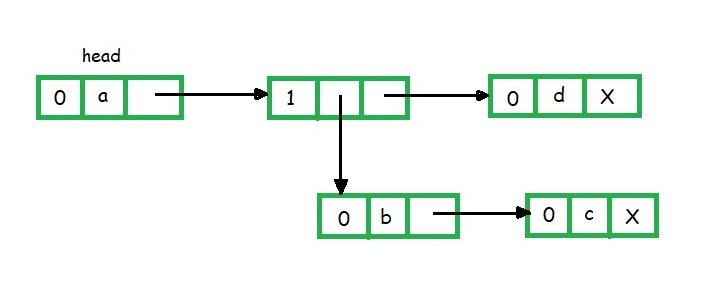When first field is 0, it indicates that the second field is variable. If first field is 1 means the second field is a down pointer, means some list is starting.

Polynomial Representation using Generalized Linked List
The typical node structure will be: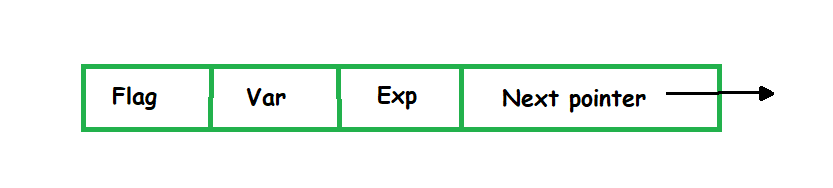• Here Flag = 0 means variable is present
• Flag = 1 means down pointer is present
• Flag = 2 means coefficient and exponent is present

Example:
9x5 + 7xy4 + 10xz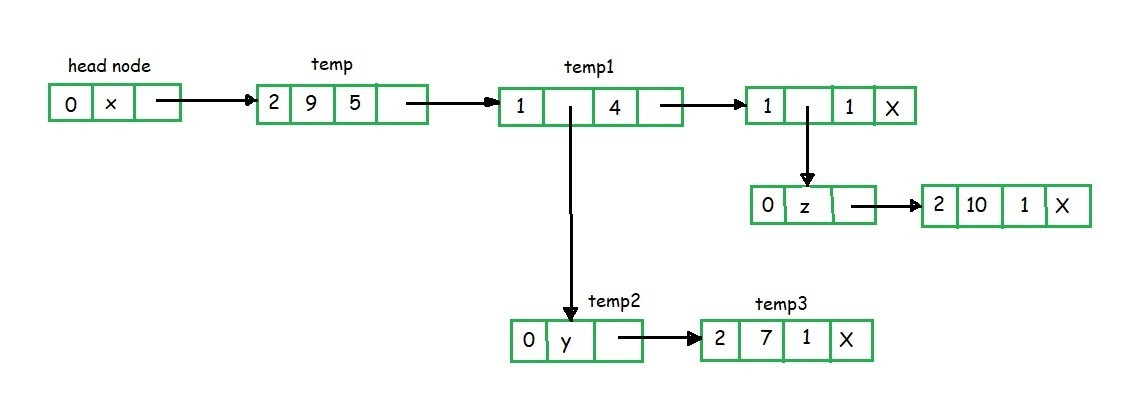In the above example the head node is of variable x. The temp node shows the first field as 2 means coefficient and exponent are present.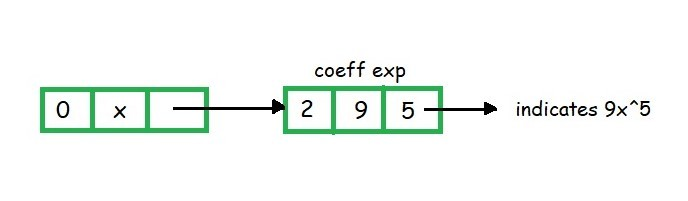Since temp node is attached to head node and head node is having variable x, temp node having coefficient = 9 and exponent = 5. The above two nodes can be read as 9x5.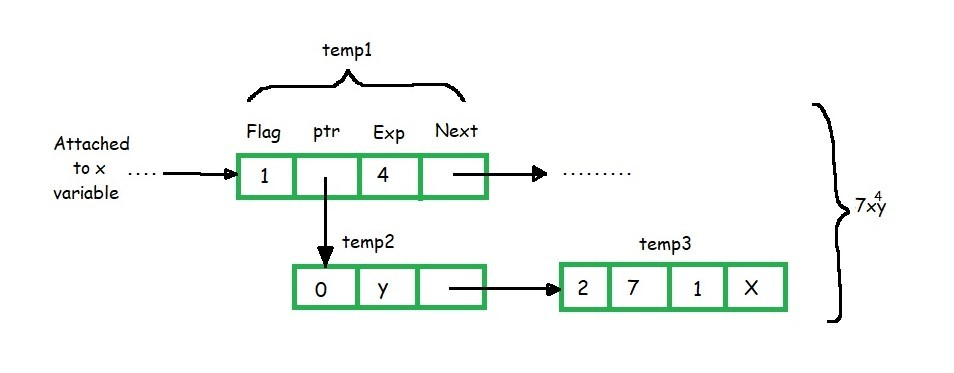Similarly, in the above figure, the node temp1 can be read as x4.

• The flag field is 1 means down pointer is there
• temp2 = y
• temp3 = coefficient = 7
• exponent = 1
• flag = 2 means the node contains coefficient and exponent values.
• temp2 is attached to temp3 this means 7y1 and temp2 is also attached to temp1 means
• temp1 x temp2
• x4 x 7y1 = 7x4y1 value is represented by above figure

My Personal Notes arrow_drop_upCheck out this Author's contributed articles.

If you like GeeksforGeeks and would like to contribute, you can also write an article using contribute.geeksforgeeks.org or mail your article to contribute@geeksforgeeks.org. See your article appearing on the GeeksforGeeks main page and help other Geeks.

Please Improve this article if you find anything incorrect by clicking on the "Improve Article" button below.

Article Tags :
Practice Tags :

Be the First to upvote.

Please write to us at contribute@geeksforgeeks.org to report any issue with the above content.